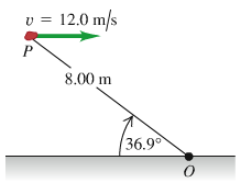# Problem: A 2.00-kg rock has a horizontal velocity of magnitude 12.0 m/s when it is at point P in the figure (Figure 1). If the only force acting on the rock is its weight, what is the rate of change (magnitude and direction) of its angular momentum at this instant?

###### FREE Expert Solution

Rate of change in angular momentum is equal to the torque on the rock.

$\overline{){\mathbf{\tau }}{\mathbf{=}}{\mathbf{r}}{\mathbf{·}}{\mathbf{F}}{\mathbf{s}}{\mathbf{i}}{\mathbf{n}}{\mathbf{\theta }}}$

F = mg

θ = (90 - 36.9)

98% (186 ratings)###### Problem Details

A 2.00-kg rock has a horizontal velocity of magnitude 12.0 m/s when it is at point P in the figure (Figure 1).If the only force acting on the rock is its weight, what is the rate of change (magnitude and direction) of its angular momentum at this instant?